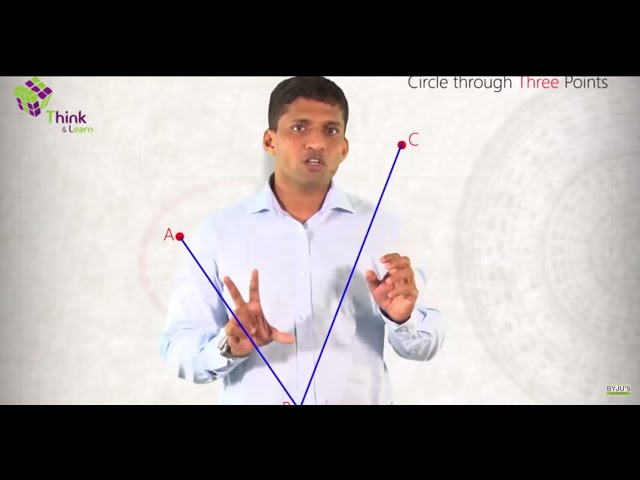# Straight Lines Class 11 Notes - Chapter 10The slope of a line (m) is determined by the value of tan θ, where, θ is the angle made by the line with the positive direction of x-axis in an anti-clockwise direction. The slope of the line passing through two points P (a1, b1) and Q (a2, b2) is given by:

$$\begin{array}{l}m\;=\;tan\;\theta \;=\;\frac{b_{2}-b_{1}}{a_{2}-a_{1}}\end{array}$$

If m1 and m2 be the slopes of two lines. The angle θ between them is given by:

$$\begin{array}{l}tan\;\theta =\;\pm \frac{(m_{1}-m_{2})}{1\;+\;m_{1}m_{2}}\end{array}$$

In case of acute angle,

$$\begin{array}{l}tan\;\theta =\;\pm \frac{(m_{1}-m_{2})}{1\;+\;m_{1}m_{2}}\end{array}$$

• For parallel lines, m1 = m2
• For perpendicular lines, m1.m2 = -1

Three points A(h, k), B(m1, n1) and C(m2, n2) are said to be collinear, if, the slope of AB = slope of BC.

i.e.

$$\begin{array}{l}\frac{n_{1}-k}{m_{1}-h}=\frac{n_{2}-n_{1}}{m_{2}-m_{1}}\end{array}$$

Or,
$$\begin{array}{l}(k-n_{1})\;(m_{2}-m_{1})\;=\;(h-m_{1})\;(n_{2}-n_{1})\end{array}$$

To get more details on Straight Lines, visit here.

### Equation of Line – Different Forms

1. The equation of a line parallel to the x-axis and at a distance (p) from x-axis is given by, y = ± p.
2. The equation of a line parallel to the y-axis and at a distance (q) from y-axis is given by, x = ± q.
3. The equation of a line [Point-slope form] having slope (m) and passing through point (a0, b0) is given by, y – b0 = m(x – a0).
4. The equation of a line [Two-point-form] passing through two points (a1, b1) and (a2, b2) is given by,

$$\begin{array}{l}y-b_{1}=\left ( \frac{b_{2}-b_{1}}{a_{2}-a_{1}} \right )(x-a_{1})\end{array}$$

5. The equation of a line [Slope intercept form] making an intercept (p) on the y-axis (slope m) is given by y = mx + p. [value of p will be +ve or -ve based on the intercept made on
the +ve or -ve side of the y-axis].

1. The equation of the line [Intercept form] making intercepts p and q on x and y-axis respectively is given by

$$\begin{array}{l}\frac{x}{a}+\frac{y}{b} =1\end{array}$$

In normal form, the equation of the line is given by x cos ω + y sin ω = p. Where, p = Length of perpendicular (p) from the origin and ω = Angle which normally makes with the +ve x-axis direction.

The points (m1, n1) and (m2, n2) are on the same or opposite side of a line px + qy + r = 0, if pm1 + qn1 + r and pm2 + qn2 + r are of the same sign or of opposite signs respectively. The lines xm1 + yn1 + o1 = 0 and xm2 + yn2 + o = 0 are perpendicular, if, m2m1 + n2n1 = 0.

### Straight Lines Class 11 Practice Questions

1. Determine the equation of lines passing through (2, 3) and making angle 60° with
x-axis.
2. Determine the equation of a line parallel to the line 2x + 5y = 2 and passing through the point of intersection of lines 12x + 2y = 35 and 4x + 53y + 68 = 0.
3. Determine the length of the triangle if the equation of the base of an equilateral triangle is 2x + 3y = 42 and the vertex is (3, -4).
1. Determine the value of p and q if the intercepts cutoff on the coordinate axes by the
line px + qy + 3 = 0 are equal but have opposite signs to those cut off by the
line x – 2y + 4 = 0 on the axes.
1. Determine the equation of the line if the intercept of a line connecting the coordinate axes is separated by a point (4, -3) in the ratio 2:4.

## Frequently asked Questions on CBSE Class 11 Maths Notes Chapter 10: Straight Lines

### What are ‘Straight lines’?

In geometry, the notion of line or straight line was introduced by ancient mathematicians to represent straight objects with negligible width and depth.

### What is ‘Distance formula’?

The distance formula to calculate the distance between two points

### What is ‘Concurrency’?

Concurrency is the concept of executing two or more tasks at the same time.